# Learn Javascript 2017, Loops, for loopsFor loops can be tough to follow if you are learning to code for the first time. This animated explanation should make it slightly easier.

For loops are a fundamental part of pretty much every language used in web development. You learn about them in the first week of every computer science 101 class, and they will be a part of any introductory online course.

Even so, if JavaScript is your first coding language (like it was for me), the concept of a for loop can still feel a little mysterious. Sure, you might understand it in principle. But once you start layering other concepts on top — like arrays, objects, and more complicated math — you might find that you don’t understand them as clearly as you would like.

So, I wanted to create a crystal-clear explanation that will be lodged in your brain. When you are introduced to more complicated concepts, it will be easy to use them inside your for loops.

#### So what are for loops?

If you aren’t already familiar, for loops allow you to take action on a list of elements without explicitly naming each element.

Say you have the following list of elements: 0,1,2,3,4. You would not want to manually plug each one into a function or access the index of an array. You would want to loop through them and automatically take the action for each element in the list. I will explain more in a moment.

#### Why the heck do I need a for loop explanation?

Let’s look at a basic one.

``````for (let i = 0; i<5; i++){
console.log(i);
}
``````

This would output:

``````0
1
2
3
4
``````

Here are the two problems that I see:

• What is the concept of i? It is used differently than other variables.
• Where does the iteration happen? In other words, when does i increase?We are going to look at for loops a different way. Imagine that you are a scuba diver, and you are planning a day-long trip to a new location. You are checking out a new reef, so you will probably want to take multiple trips to the bottom to make sure you see all the coral and marine life.

#### Preparing for the dive (initialization and condition)

``````for (let i = 0; i<5; i++){
console.log(i);
}
``````

Before you start your dive, you need to determine how many oxygen tanks you will need over the course of the day.

let i= 0;

This is the initialization. Ittells you the value of the first oxygen tank. In this case, you start at tank 0.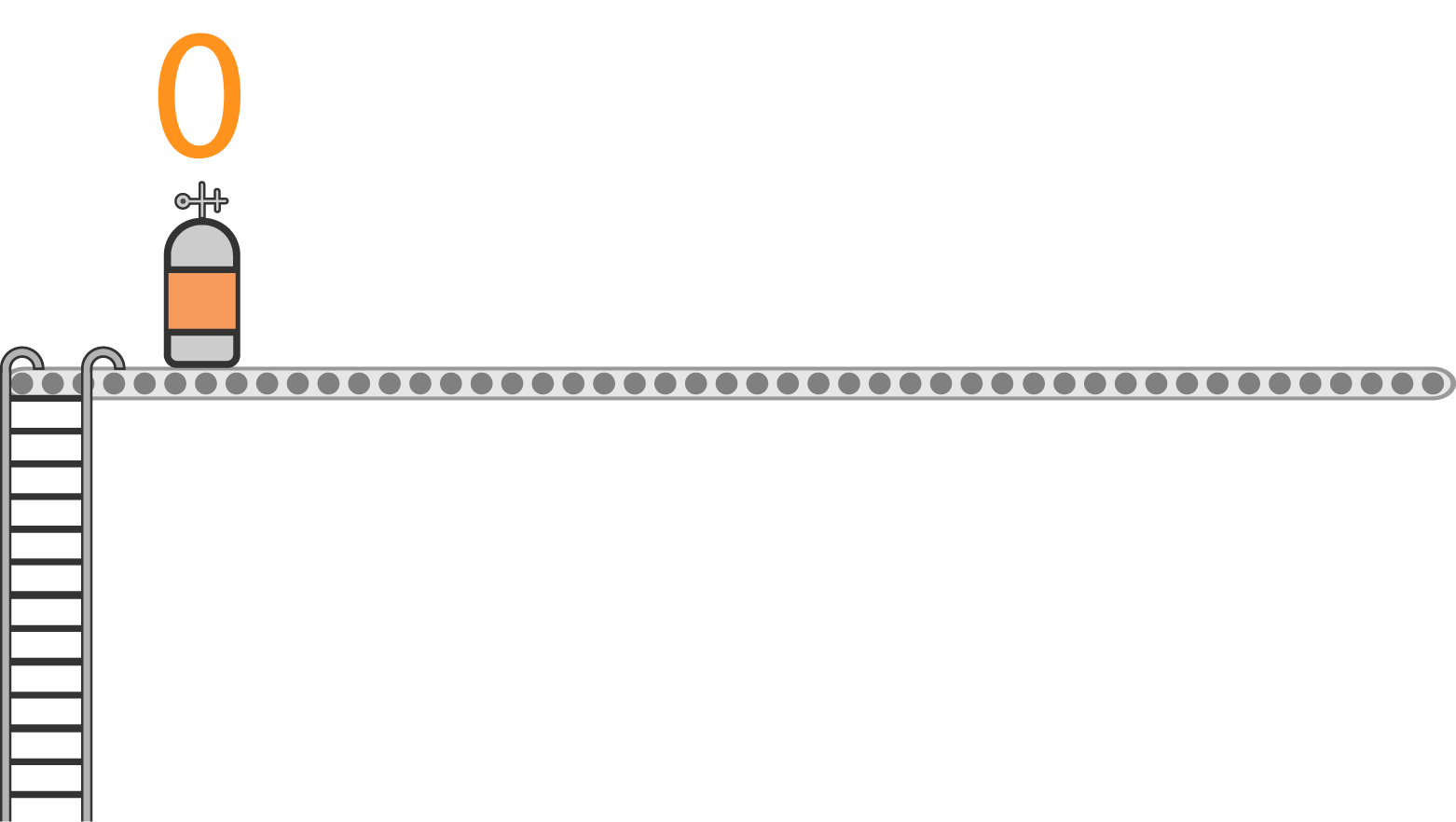i < 5

This is the condition. It is kind of like the capacity of the boat. You can only add as many oxygen tanks as your boat will hold.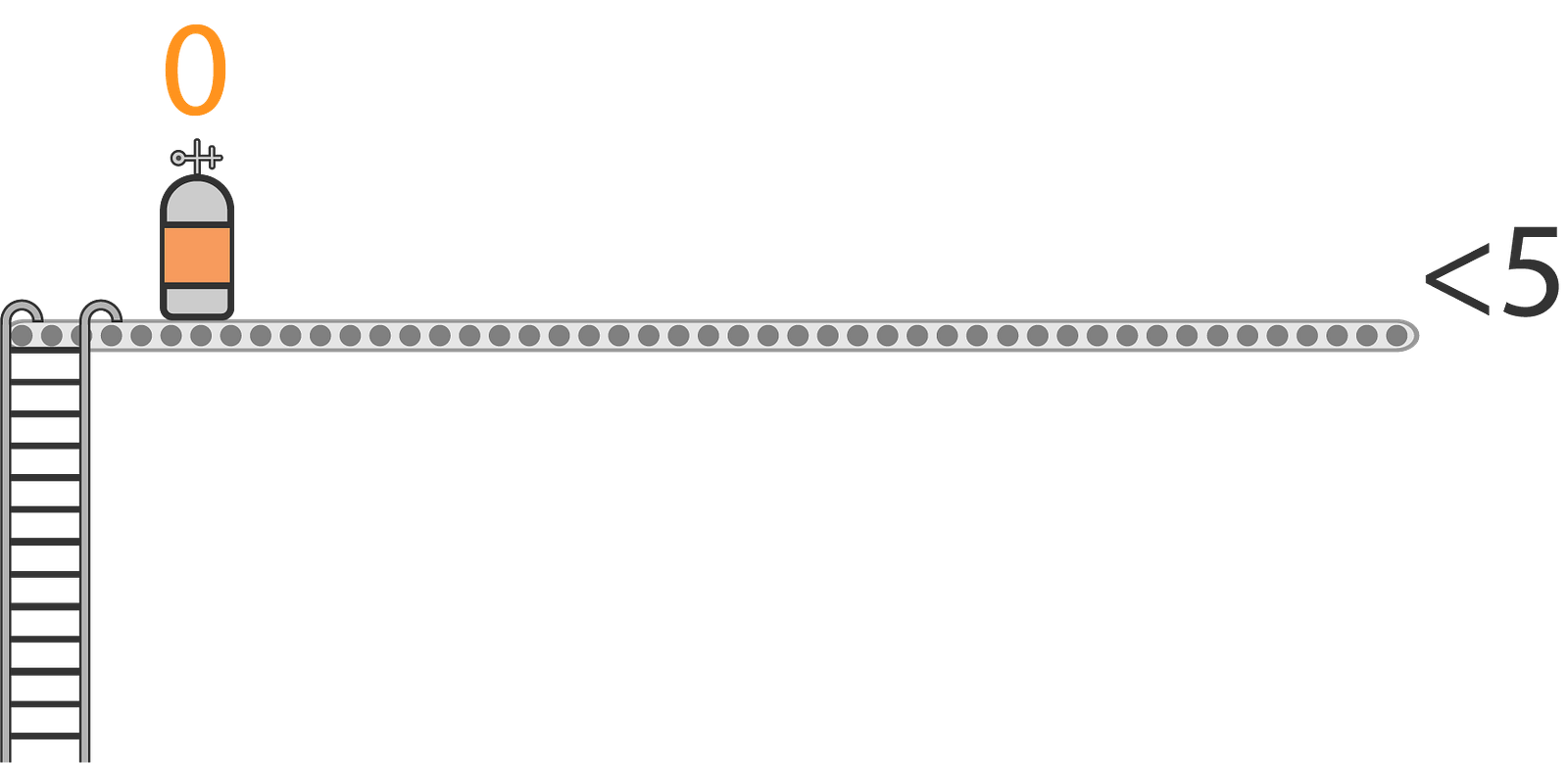#### Setting up oxygen tanks (update)

So far, we know that our first oxygen tank has a value of 0, and the last one must be less than 5. But how many tanks do you need to prepare?

The last part, called the update, tells us how many tanks we need to line up.

i++

This is shorthand for: i = i+1

It means that every time we finish a loop, we will add 1 to i. Since i starts at 0, here is what that looks like.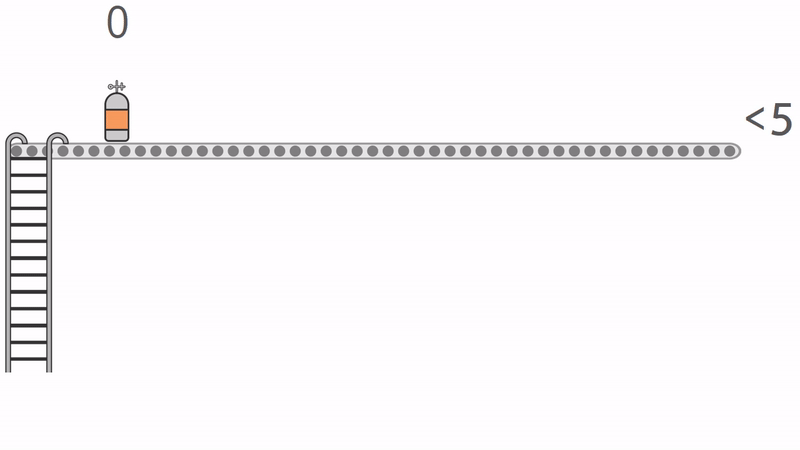We continue to add oxygen tanks until we hit the limit. When we add one tank at a time, the last value that fulfills the condition is 4.

#### Scuba diver goes on first trip (iteration)

So far, we know the start value of i (0) and each value of i that fulfills the condition (0–4). We are all set up. Now, we need to bring in the scuba diver and execute the statements within the for loop.

Imagine that we are running this loop:

``````for (let i = 0; i<5; i++){
console.log("The current value is: " + i);
}
``````

So, your scuba diver starts at a value of 0.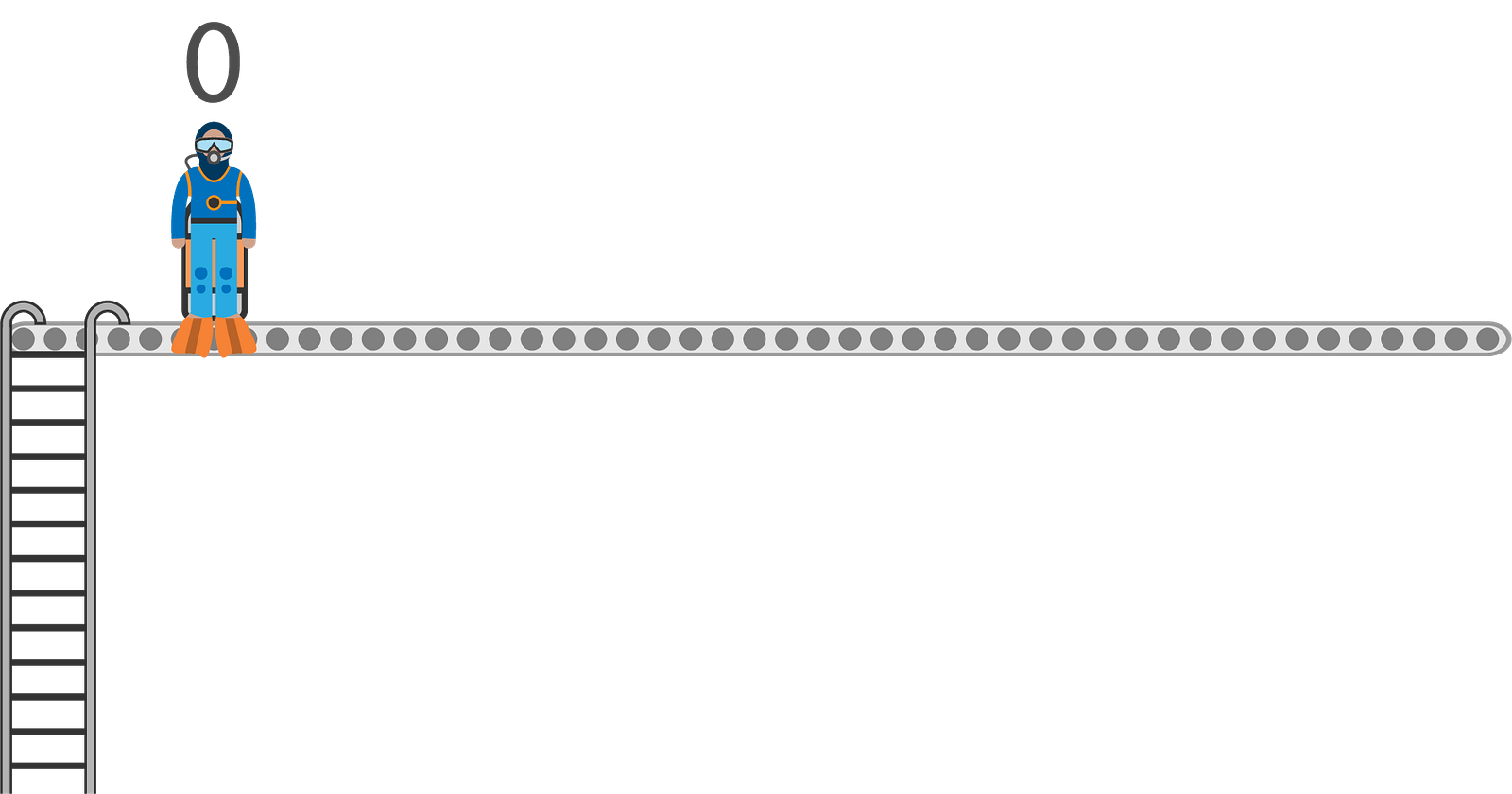Do you see where we are going with this? Your scuba diver is actually i! And it is going to move through the contents of the for loop, and then come up for another tank.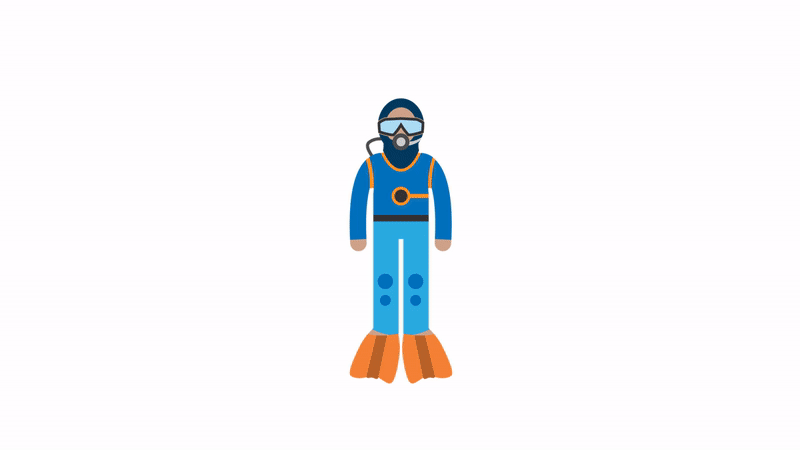Right now, the loop only has one statement. Here is what will happen on the first iteration.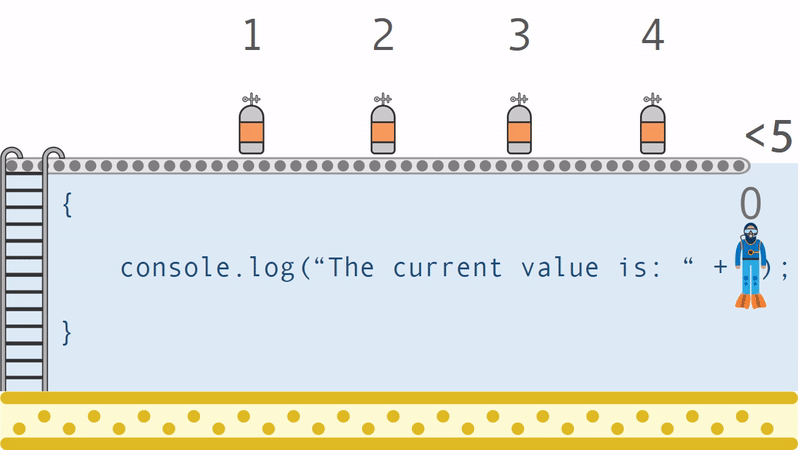The console would log: “The current value is 0” since i is 0. Your scuba diver carries the value of each oxygen tank as it moves through the array.

#### Swimming back up (second iteration)

``````for (let i = 0; i<5; i++){
console.log("The current value is: " + i);
}
``````

Since this for loop only has one statement, you just completed the first iteration. Now, you need to run through it using the next value.

You will usually find that your for loops have many lines of code. But for the time being, we are just sticking to the one line so that you can trace the path of i.

Here’s what happens when you hit that last bracket: }.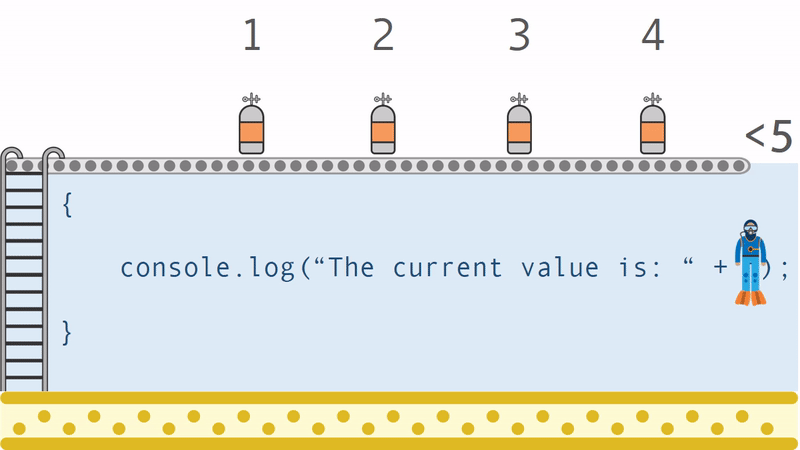The scuba diver drops the 0 tank and climbs the ladder back up to grab the second tank, with a value of 1. The scuba diver is doing some sort of weird solitary relay race, but hey, that is the nature of a for loop. You want it to be as quick as possible. Now, the diver is ready to go through the loop again with a value of 1.

#### The rest of the oxygen tanks (every iteration)

Now the diver needs to take each oxygen tank through the loop until they are all gone.

Each time, we will log a new statement to the console.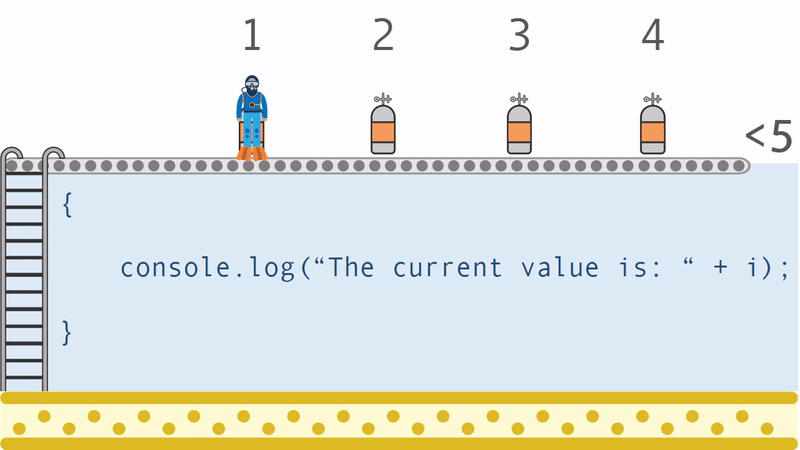The final output would be:

``````The current value is 0
The current value is 1
The current value is 2
The current value is 3
The current value is 4
``````

By the end of the loop, i is equal to 4 and cannot go any higher due to the condition, so the loop is done. If you run the loop again, i will start at 0 again due to the initialization.

Why aren’t there multiple scuba divers? Because there is only one i! There can only be one value of i going through the loop at any time. i needs to climb back up to the top to grab the next value.

#### Changing the conditions

Let’s say that now, instead of counting up from 0 to 5, you want to count down every whole number from 10 to 2. How might you do that?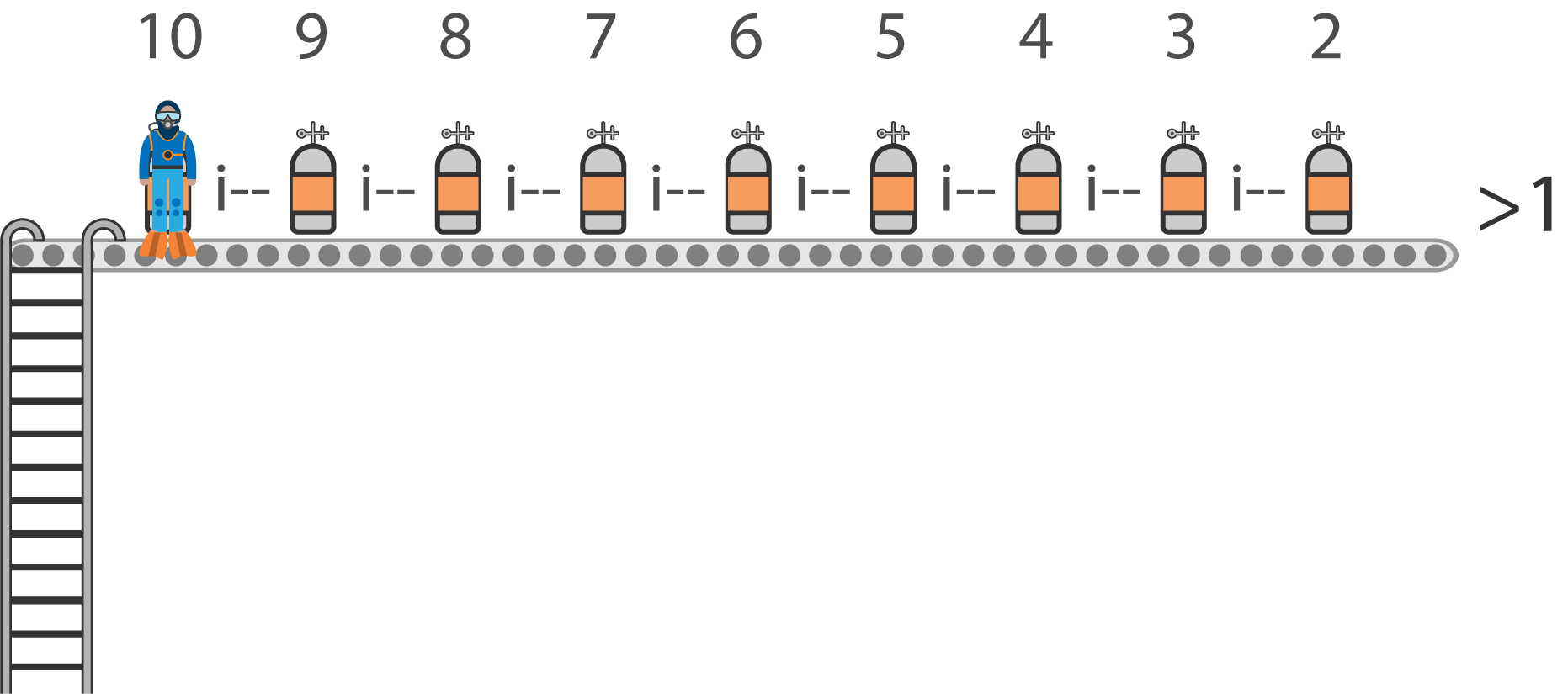Since the start value is 10, you need to initialize i to 10.

let i= 10;

Then, since you want the last number to be 2, you need to set a condition for all numbers greater than 1.

i > 1

And, instead of i++, we use i - -, which is equivalent to i= i-1.

Full code:

``````for ( let i = 10; i > 1; i --){}
``````

#### Using for loops alongside arrays

Before you read this section, you should understand arrays. If you have not studied them before, check out my guide here.

For loops are commonly used to iterate through arrays. Let’s say you have an array full of test scores.

``````var testScores = [64, 86, 73, 82, 95, 62, 87, 99];
``````

You want to log a message related to each score in the console. As you go through your loop, you need to be able to align the current value of i with the index of the array. Therefore, you need to make sure the for loop goes through every item in the array, no matter how many test scores are in it.

We can use the length property of the array to discover how many elements it contains. In this case, it is 8. Remember that arrays are also 0-indexed, meaning the first element of the array has an index of 0.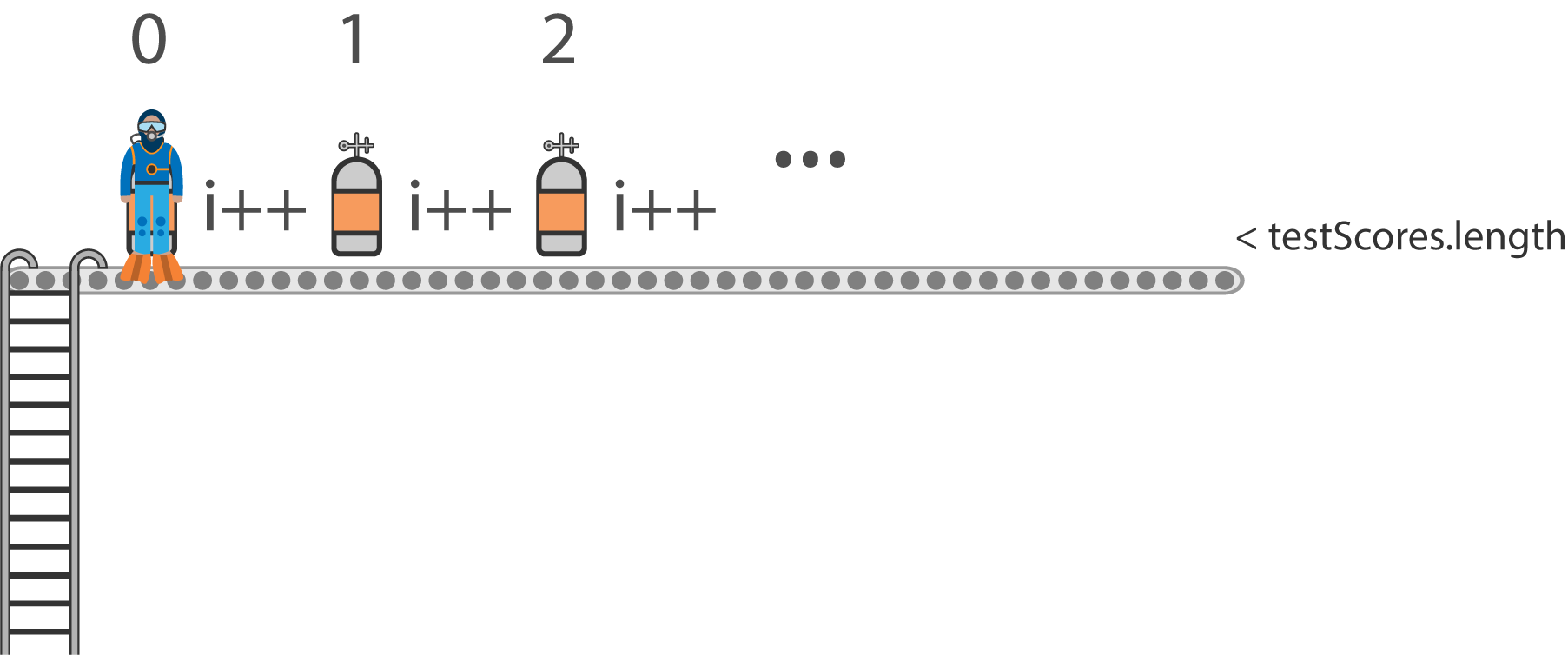We initialize i to 0. We can actually use the testScores.length in the condition, so that it will work no matter how many elements are in the array.

i< testScores.length

We can reference each item in the array by using i as the index.

testScores[i]

Back to our scuba diver: It needs to takes as many trips as there are elements in the array. This is why it is so important for us to track i. In this example, the values of the tanks map to specific items in the array.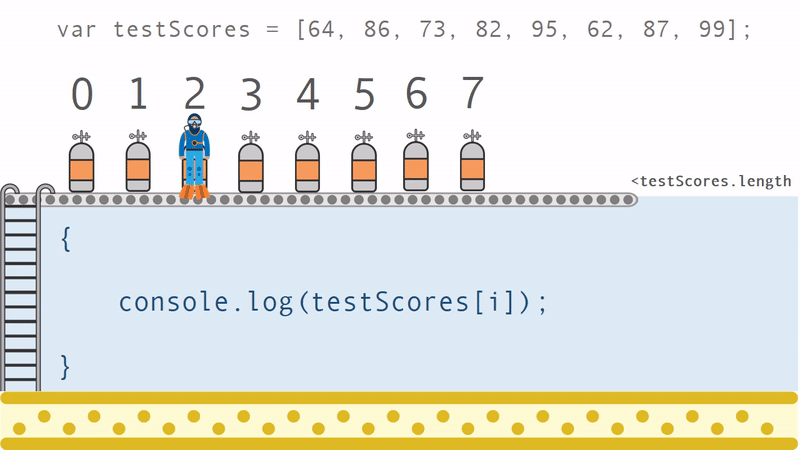The example above shows the third iteration of the loop, which will access the third element of the array at index 2. See how that can be tricky?

Here is the final code for that:

``````for (let i=0; i< testScores.length; i++){
console.log(testScores[i]);
}
``````

**Recommended Courses: **

A Simple Node.js/Mongo/Restify API in Less Than 3 Hours
https://goo.gl/gcsxof

Create Fast, Scalable Network Applications with Familiar Javascript Code
https://goo.gl/7924MS

Essentials in JavaScript ES6 - A Fun and Clear Introduction
https://goo.gl/g5gkq7

Vue JS 2.0 - Mastering Web Apps
https://goo.gl/FYCU3J